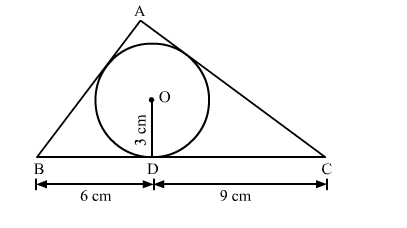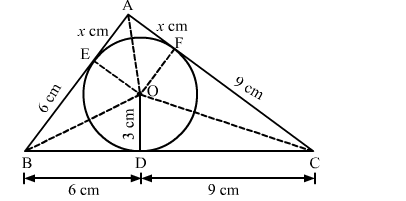# In the given figure, a triangle ABC is drawn to circumscribe a circle of radius 3 cm such that the segments BD and DC`
Question:

In the given figure, a triangle ABC is drawn to circumscribe a circle of radius 3 cm such that the segments BD and DC into which BC is divided by the point of contact D are, of lengths 6 cm and 9 cm respectively. If the area of △ABC = 54 cm2 then find the lengths of sides of AB and AC.Solution:

Construction: Join OA, OB, OC, OE ⊥ AB at E and OF ⊥ AC at FWe know that tangent segments to a circle from the same external point are congruent.
Now, we have
AE = AF, BD = BE = 6 cm and CD = CF = 9 cm
Now,

Area $(\triangle \mathrm{ABC})=$ Area $(\triangle \mathrm{BOC})+$ Area $(\triangle \mathrm{AOB})+$ Area $(\triangle \mathrm{AOC})$

$\Rightarrow 54=\frac{1}{2} \times \mathrm{BC} \times \mathrm{OD}+\frac{1}{2} \times \mathrm{AB} \times \mathrm{OE}+\frac{1}{2} \times \mathrm{AC} \times \mathrm{OF}$

$\Rightarrow 108=15 \times 3+(6+x) \times 3+(9+x) \times 3$

$\Rightarrow 36=15+6+x+9+x$

$\Rightarrow 36=30+2 x$

$\Rightarrow 2 x=6$

$\Rightarrow x=3 \mathrm{~cm}$

$\therefore \mathrm{AB}=6+3=9 \mathrm{~cm}$ and $\mathrm{AC}=9+3=12 \mathrm{~cm}$